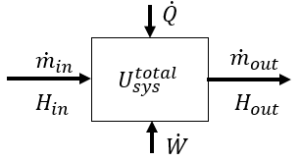LearnChemE

#### First Law - Open Systems: Screencast

Explains the terms in the first law of thermodynamics for systems with mass flow into and/or out of the system and looks at some special cases.

We suggest that after watching this screencast, you list the important points as a way to increase retention.

Introduces the concept of flow work and derives the equations governing it.

We suggest that after watching this screencast, you list the important points as a way to increase retention.

Optional screencast: Energy Balance Around a Turbine

##### Important Equations:Energy balance (ignoring kinetic and potential energies):

$\frac{dU_{sys} ^{total}}{dt} = \dot{m}_{in} H_{in} – \dot{m}_{out} H_{out} + \dot{Q} + \dot{W}$

where $$U_{sys} ^{total}$$ is the total internal energy of the system at anytime (kJ)
$$H_{in}$$ and $$H_{out}$$ are specific enthalpies (kJ/kg)
$$\dot{m}$$ is a mass flow rate (kg/s)
$$\dot{Q}$$ is heat added per time (kJ/s)
$$\dot{W}$$ is work added per time (kJ/s) and it includes both shaft work and expansion/compression work.

For a system with no outlet stream and an inlet stream of constant enthalpy, the integrated for of the first law is:

$m_f U_f – m_i U_i = (m_f – m_i)H_{in} + Q^t + W^t$

where $$m_i$$ is the mass in the system at $$t = 0$$
$$m_f$$ is the mass in the system at time $$t$$
$$U_i$$ is the specific internal energy (kJ/kg) in the system at $$t=0$$
$$U_f$$ is the specific internal energy (kJ/kg) in the system at time $$t$$
$$H_{in}$$ is the specific enthalpy (kJ/kg) of the feed stream
$$Q^t$$ is the heat added in time $$t$$ (kJ)
$$W^t$$ is the work added in time $$t$$ (kJ)
Therefore $$m_f – m_i$$ is the total mass added to the system.

Steady-state adiabatic compressor or turbine: $\dot{m}_{in} H_{in} + \dot{W}_S = \dot{m}_{out} H_{out}$ where $$\dot{W}_S$$ is the shaft work per time

Throttle:   $H_{in} = H_{out}$

Adiabatic reversible expansion or compression (open or closed system) for an ideal gas:

$\frac{T_2}{T_1} = \left( \frac{P_2}{P_1} \right) ^\frac{R}{C_P}$